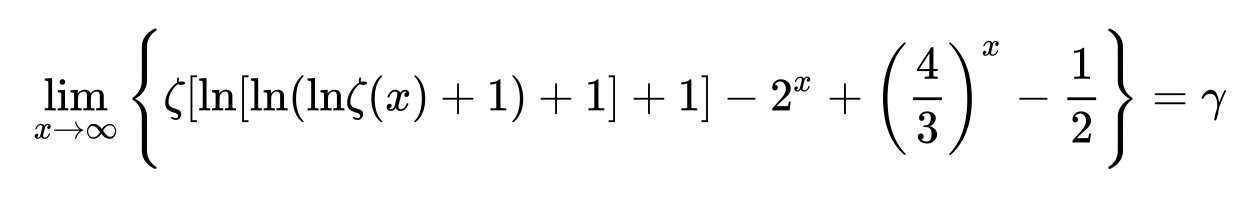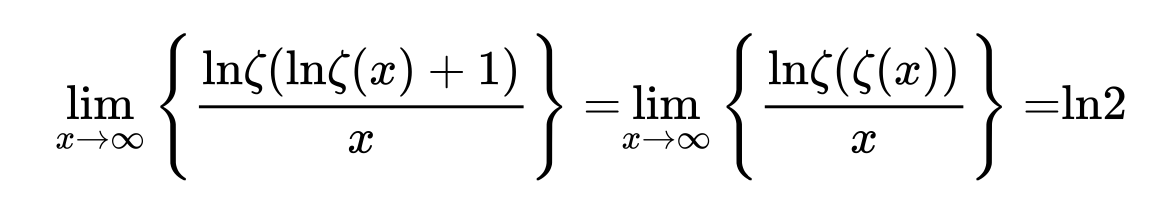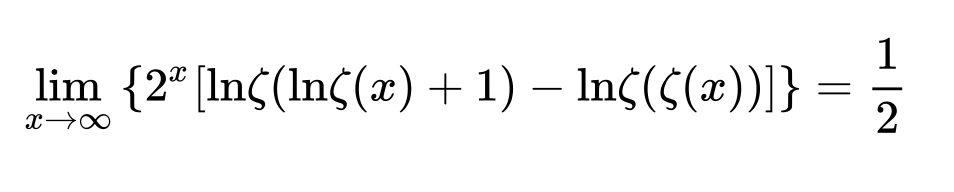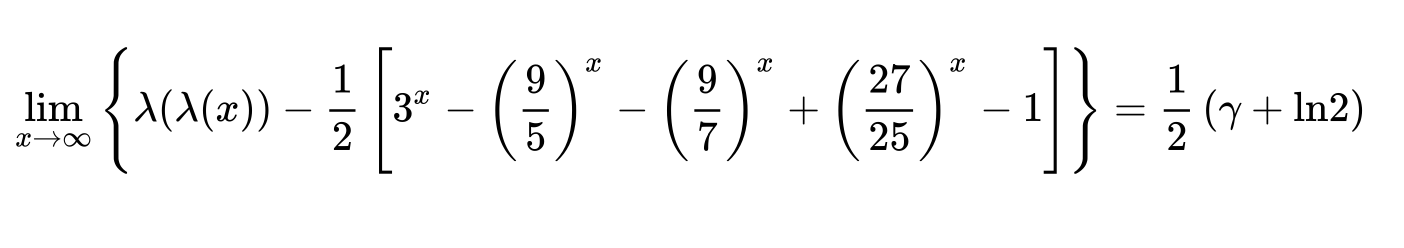# A new way of approaching the pole of the Riemann zeta function - and a new conjectured formula

On the Wolfram page about the Euler-Mascheroni Constant $$\gamma$$, the following amazing limit is given without proof (referring to "personal communication"):

$$\lim_{z\to\infty}\left[\zeta(\zeta(z))-2^z+\Bigl(\frac43\Bigr)^z\right]=\gamma -1$$ I have checked to thousands of decimals that likewise $$\lim_{z\to\infty}\left[\zeta\Bigl(\frac1{\zeta(z)}\Bigr)+2^z-\Bigl(\frac43\Bigr)^z\right]=\gamma$$ and started wondering what happens if we combine both, e.g. by fixing a weight $$0\leqslant\lambda\leqslant1$$ and looking at the behaviour of $$f_\lambda(z):=\zeta \Bigl(\lambda{\zeta(z)+\frac{1-\lambda}{\zeta(z)}}\Bigr)$$ for again growing $$z$$ on the real axis. So the argument in the big parenthesis still tends to $$1$$, such that the pole at $$\zeta(1)$$ is approached in different ways depending on $$\lambda$$. And something cute happens: Numerically it looks like $$\boxed{\color{blue}{ \lim_{z\to\infty}\left[f_{\lambda}(z)- \frac1{2\lambda-1}\left(2^z- \Bigl(\frac43\Bigr)^z\right) \right]=\gamma -\frac{\lambda}{(2\lambda-1)^2}}}$$ for $$\lambda\ne\frac12$$ (even outside the interval $$[0,1]$$), while, completely off, $$f_{1/2}(z)\sim 2\cdot4^z-4\cdot\Bigl(\frac83\Bigr)^z -2\cdot 2^z -\text {(5 other exp terms)}-4+\gamma.$$

I am wondering if such a formula has appeared before and how to prove it!

• A nice consequence of your conjectured formula is $\displaystyle{\lim_{z\to\infty}(f_{\lambda}(z)+f_{1-\lambda}(z))=\gamma-\frac{1}{(2\lambda-1)^2}}$. Dec 1, 2019 at 20:38

The paper entitled Euler constant as a renormalized value of Riemann zeta function at its pole by Andrei Vieru contains a derivation of the first formula in the OP (Benoȋt Cloitre's formula), and a method to obtain variations thereof, such asas well as similar iterates in terms of the Dirichlet function $$\lambda(s)=\sum_{n=0}^\infty(2n+1)^{-s}$$,The first formula is trivial. $$f(s)= \frac1{s-1}+\gamma +O(s-1)$$ $$g(z)=1+2^{-z}+3^{-z}+4^{-z}+O(5^{-z})=1+2^{-z}(1+(3/2)^{-z}+(4/2)^{-z}+O(5/2)^{-z})$$

$$f(g(z)) = \frac1{2^{-z}(1+(3/2)^{-z}+(4/2)^{-z}+O(5/2)^{-z})}+\gamma+O(2^{-z})$$ $$=2^z \left(1-(3/2)^{-z}-(4/2)^{-z}+O(5/2)^{-z}+(O(3/2)^{-z})^2\right)+\gamma + O(2^{-z})$$

$$=2^z- (3/4)^{-z}-1+O(9/8)^{-z}+\gamma$$

• I would not call it exactly "trivial"... Pulling out the $2^{-z}$ is rather clever than trivial. Could we agree on "straightforward"? Dec 2, 2019 at 9:02
• It doesn't contain any non-trivial information about $\zeta(s)$ (for example the abscissa of convergence of $\frac1{\zeta(s)-1}$ is non-trivial) Dec 2, 2019 at 10:40
• yes I agree. But sometimes one can make sophisticated proofs out of trivial information/axioms, think of some of the proofs of $\zeta(2)=\frac{\pi^2}6$. :) :) Dec 2, 2019 at 10:49
• For $\lambda=1/2$, this approach shows that there are altogether $8$ exponential terms. I have not included in the edit those with $(\frac{16}{9})^z,(\frac{16}{10})^z,(\frac{16}{12})^z,(\frac{16}{14})^z,(\frac{16}{15})^z$. Doing their coefficients manually is too error-prone... Dec 2, 2019 at 12:34
• Yes I tried in pari-gp but I didn't success in manipulating $2^{-z}+O(3^{-z})$ (whereas $x+O(x^2)$ is a built-in function) Dec 4, 2019 at 14:00